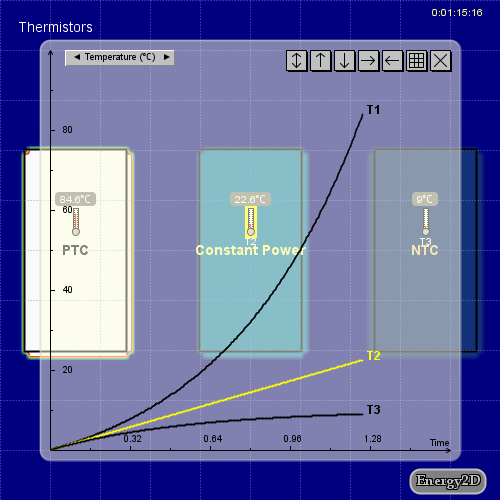## Simulating PTC and NTC heating elements with Energy2DFigure 1: A demo simulation.

A heating element converts electricity into heat through Joule heating: Electric current passing through the element encounters resistance, causing the temperature of the element to rise. A thermistor is a type of resistor whose resistance changes significantly with temperature. In a heating element that uses a thermistor with a positive temperature coefficient (PTC), called a PTC heating element, the temperature increases rapidly. In a heating element that uses a thermistor with a negative temperature coefficient (NTC), called a NTC heating element, the heating will gradually weaken when the temperature increases.Figure 2: Setting the temperature coefficient.

Several Energy2D users have requested adding PTC/NTC controls to the software. So this was added last night. You can now set the temperature coefficient while defining a power source, as shown in Figure 2.

Figure 1 shows the comparison of the temperature increasing in a PTC heater, a constant-power heater, and a NTC heater, with the temperature coefficients being 0.1, 0, and -0.1, respectively. Note that in the case of constant power, the temperature increases linearly in time (as per the definition of constant power), whereas PTC and NTC exhibit nonlinear behaviors.

You can click the link under the image to run the simulation yourself.

## 3 thoughts on “Simulating PTC and NTC heating elements with Energy2D”

1.Shannon Willard says:

This is very misleading (or plain incorrect) and would only be correct for a constant current source, which is a pretty unusual occurrence.
As a PTC heater gets hotter, the electrical resistance increases, reducing the output power (for a normal fixed voltage power supply). If the PTC is cooled (i.e. by blowing lower temperature air over the device, as would happen in a fan forced heater) the resistance lowers again, current increases and power increases until the conditions change (i.e. the fan speed reduces and the ambient air temperature increases), then the current reduces as a result of the increasing electrical resistance and power output drops, thus resulting in somewhat self regulation of the PTC temperature for a fixed voltage and environmental conditions. This is why PTCs are often used as heaters and current limiting protection devices.
The opposite is true for NTC thermistors, which is why they are typically used to limit inrush current and similar functions, because they are high(er) electrical resistance when cool and low(er) electrical resistance when hot.

2.Oleg says:

Good post. I’m going to get a Ntc thermistor from pixsys, meanwhile I’ll do some simulations.

1.Ana says: